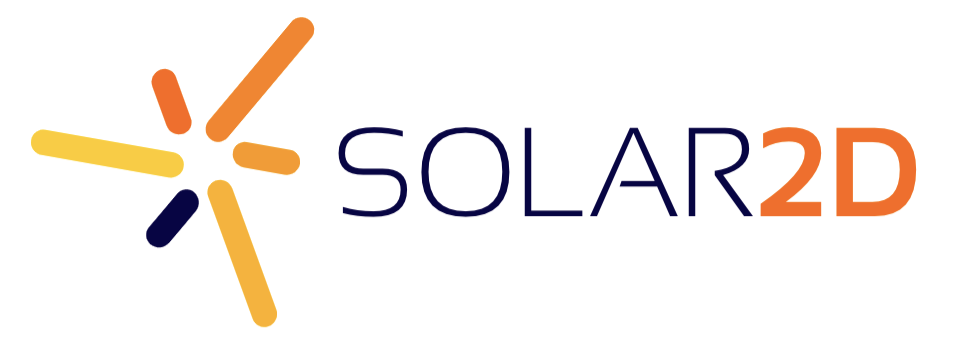# Help With Buttons and SetGravity

I am new to Corona and just starting to learn it. I was trying to build a test app with my new-found knowledge of buttons, in which you press a button to toggle the gravity on a ball, but it doesn’t work and I have no idea why. Please Help. Thank you in advance!

Here is my code:

physics = require(“physics”)
widget = require(“widget”)
physics.start()

local gravSet = false;
physics.setGravity(0,0)

local bubble = display.newCircle(300,0,10,10)

function ToggleGravity()

if gravSet == true then
gravSet = false
gravBtn:setLabel(“Start”)

``````physics.setGravity(0,0)
print("Gravity Stopped!")
``````

elseif gravSet == false then
gravSet = true
gravBtn:setLabel(“Stop”)

``````physics.setGravity(0,10)
print("Gravity Started!")
``````

end

end

gravBtn = widget.newButton({
label = “Start”,
width = 200,
height = 50,
top = 0,
left = 0,
defaultFile = “Buttons/Button1/default.png”,
overFile = “Buttons/Button1/over.png”,
onRelease = ToggleGravity
})

Because to optimize the number of mathematical operations, bodies can fall asleep.
You can prevent this from happening globally by starting the physical engine with:

``````physics = require("physics")
widget = require("widget")
physics.start(true)
``````

alternatively (and in my opinion it is better to do so) you can tell the individual object not to “fall asleep” with:

``````local bubble = display.newCircle(300,0,10,10)
``````physics.setGravity(0,0)